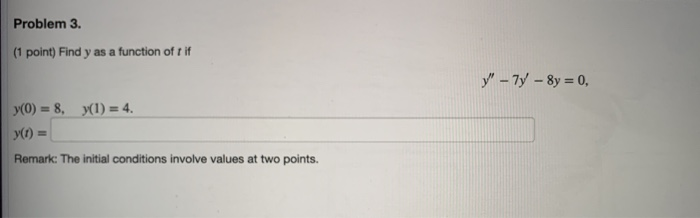# Problem 3. (1 point) Find y as a function of tif y" - 7 - 8y...

###### Question:Problem 3. (1 point) Find y as a function of tif y" - 7 - 8y = 0, y(O) = 8, y(1) = 4. y) = Remark: The initial conditions involve values at two points.